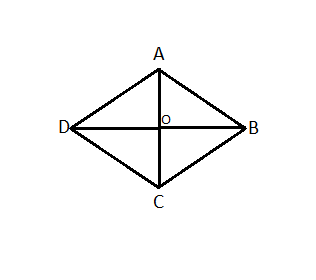Courses
Courses for Kids
Free study material
Offline Centres
MoreLast updated date: 01st Dec 2023
Total views: 381.9k
Views today: 10.81k

# Show that if the diagonals of a quadrilateral bisect each other at right angles, then it is a rhombus.Verified
381.9k+ views
Hint:
In order to solve this problem, draw the diagram of quadrilateral then join its diagonals. Apply the rules of congruency of triangles among two of the triangles formed then prove the sides are equal to show it’s a rhombus. Doing this will give you the solution.

Draw a quadrilateral ABCD and join AC and BD.Form the diagram we consider triangles AOB and AOD
It is given that diagonals bisect each other at 90 degrees.
Therefore, $\angle {\text{AOD}} = \angle {\text{AOB}}$(Right angles)
DO=OB (O is the midpoint)

AO=AO  (Common side)

So, from side angle and side (SAS) rule we can say that:
$\Delta {\text{AOD}} \cong \Delta {\text{AOB}}$
Then we can say that:
Now, we consider triangles AOB and BOC
It is given that diagonals bisect each other at 90 degrees.
Therefore, $\angle {\text{BOC}} = \angle {\text{AOB}}$(Right angles)
CO=OA (O is the midpoint)

BO=BO  (Common side)

So, from side angle and side (SAS) rule we can say that:
$\Delta {\text{BOC}} \cong \Delta {\text{AOB}}$
Then we can say that:
BC=AB ……(2)
Now, we consider triangles COD and BOC
It is given that diagonals bisect each other at 90 degrees.
Therefore, $\angle {\text{BOC}} = \angle {\text{COD}}$(Right angles)
BO=OD (O is the midpoint)

CO=CO  (Common side)

So, from side angle and side (SAS) rule we can say that:
$\Delta {\text{BOC}} \cong \Delta {\text{COD}}$
Then we can say that:
BC=CD ……(3)
So, from (1),(2) and (3) we can say that,